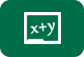# Free Algebra WorksheetsTutor-USA offers a great collection of Free Algebra Worksheets that are 100% free for use by students, teachers, home school parents or anyone using them in an educational setting. All free algebra worksheets are formatted for printing and are perfect for use in the classroom, for algebra homework assignments, or by students for extra practice or for help in studying for an exam. Many include answer keys.

Teachers

Save some time and create a class or homework assignment using our free worksheets.

Students

Practice makes perfect. Our algebra worksheets are great resource for finding extra problems to complete while studying for an exam or for further understanding of a homework assignment.  Many of our worksheets contain answer keys so you can check your work immediately.

Homeschool Parents

Our Algebra worksheets are a great addition to any home school math curriculum.

Below you can find our collection of algebra worksheets and resources. Regardless of the grade or topic you are working on, you are sure to find an appropriate worksheet for the lesson.

You can also browse Algebra Worksheets by Topic

In this free worksheet, students must use the coordinate plane to answer questions such as "In which quadrant does the point lie?", "Find the value of k if (k, 7) lies on the line y =...
Worksheet (Pre-Algebra)
Problems: 8
In this free algebra worksheet, students must factors polynomials expressions.
Worksheet (Pre-Algebra)
Problems: 15
In this free algebra printable, students must rationalize the denominator of fractions by rewriting the fractions so they form a new fraction that is equivalent to the original with a rational...
Worksheet (Algebra)
Problems: 10
In this free math worksheet, students must solve problems on geometric progressions. Problems require students to identify terms of geometric progressions and geometric series, to find missing...
Worksheet (Geometry)
Problems: 12
This free probability worksheet contains problems on combinations. Students must use the theorem for combinations to calculate combinations and solve counting problems.
Worksheet (Algebra)
Problems: 13
This free probability worksheet contains problems on permutations. Students must solve counting related problems using the theorem for permutations.
Worksheet (Algebra)
Problems: 10
This free algebra worksheet contains problems on matrices. Students must find the product of matrices, write matrix equations, and solve matrix equations.
Worksheet (Algebra)
Problems: 9
In this free math worksheet, students must divide and simplify fractions and mixed numbers. All problems contain only numbers (no variables).
Worksheet (Pre-Algebra)